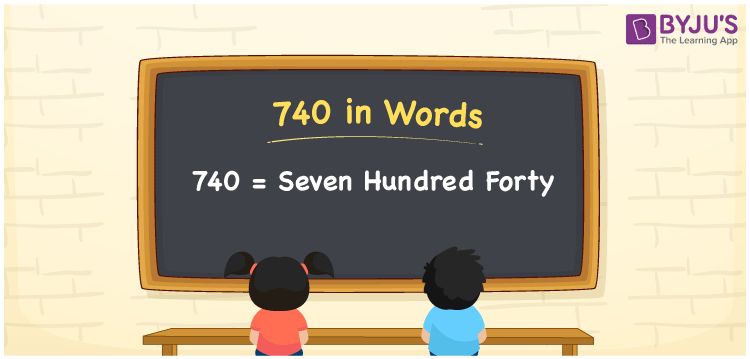# 740 in Words

740 in words is written as Seven hundred forty. In both the International System of Numerals and the Indian System of Numerals, 740 is written as Seven hundred forty. The number 740 is a Cardinal Number as it represents some quantity. For example, the “cost of the shirt is 740 rupees”.

 740 in Words Seven hundred forty Seven hundred forty in Number 740

## 740 in English Words

We write 740 in English Words using the letters of the English alphabet. Therefore, we read 740 in English as “Seven hundred forty”.## How to Write 740 in Words?

To write 740 in words, we shall use the place value chart. In the place value chart, write 7 in the hundreds, 4 in the tens, and 0 in the ones, respectively. Now let us make a place value chart to write the number 740 in words.

 Hundreds Tens Ones 7 4 0

Thus, we can write the expanded form as

7 × Hundred + 4 × Ten + 0 × One

= 7 × 100 + 4 × 10 + 0 × 1

= 700 + 40 + 0

= 740

= Seven hundred forty.

740 is a natural number, the successor of 739 and the predecessor of 741.

740 in words – Seven hundred forty

• Is 740 an odd number? – No
• Is 740 an even number? – Yes
• Is 740 a perfect square number? – No
• Is 740 a perfect cube number? – No
• Is 740 a prime number? – No
• Is 740 a composite number? – Yes

## Frequently Asked Questions on 740 in Words

Q1

### How to write 740 in words?

740 in words is written as Seven hundred forty.
Q2

### How to write 740 in the International and Indian System of Numerals?

In both, the system of numerals, 740 in words, is written as Seven hundred forty.
Q3

### 740 comes between which two natural numbers?

740 comes between 739 and 741.